# Perpendicular Line

Perpendicular Line

## Table of Contents

 1 Definition of Perpendicular 2 Perpendicular Lines 3 Tips and Tricks 4 How to Draw Perpendicular Lines? 5 Properties of Perpendicular Lines 6 Solved Examples on Perpendicular 7 Challenging Questions on Perpendicular 8 Practice Questions on Perpendicular 9 Maths Olympiad Sample Papers 10 Frequently Asked Questions (FAQs) 11 FREE Worksheets on Perpendicular Lines

We at Cuemath believe that Math is a life skill. Our Math Experts focus on the “Why” behind the “What.” Students can explore from a huge range of interactive worksheets, visuals, simulations, practice tests, and more to understand a concept in depth.

Book a FREE trial class today! and experience Cuemath's LIVE Online Class with your child.

## Definition of Perpendicular

A perpendicular is a line that makes an angle of $$\mathbf{90^{\circ}}$$ with another line.

$$90^{\circ}$$ is also called a right angle.

Example of a perpendicular line: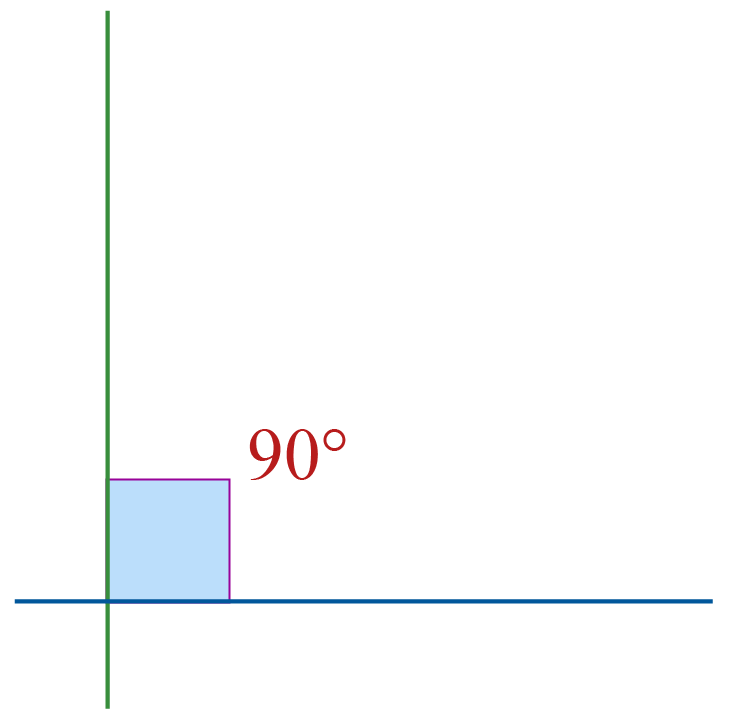Here, the blue line and the green line are perpendicular to each other.

Examples of lines that are not perpendicular: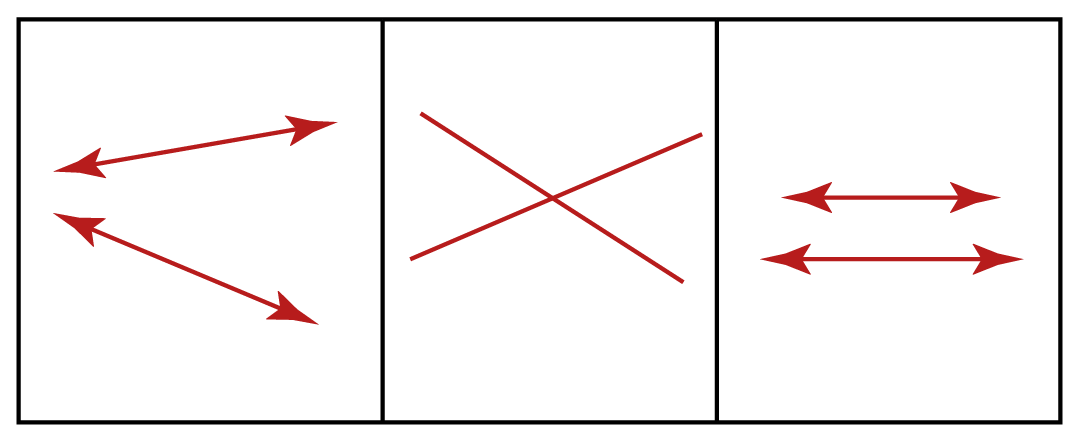Here, in each example, the angle between the two lines is NOT $$90^{\circ}$$

Hence, they are NOT perpendicular.

## Perpendicular Lines

Perpendicular lines in math are two lines where the angle between them is $$90^{\circ}$$

The symbol used in between the two lines to say that they are perpendicular is $$\mathbf{\perp}$$

If two lines $$\overline{AB}$$ and $$\overline{CD}$$ are perpendicular to each other, we represent it using the notation $$\mathbf{ \overline{AB} \perp \overline{CD}}$$.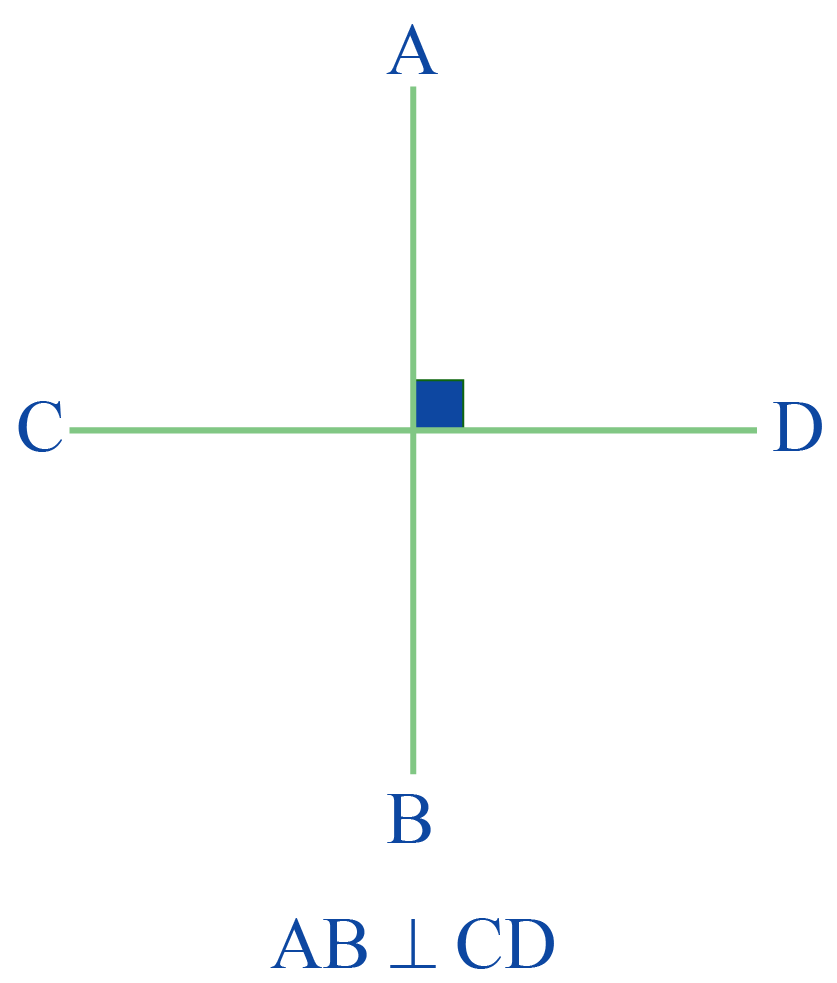Perpendicular shapes in geometry refer to shapes that include perpendicular lines.

Some perpendicular shapes in geometry are: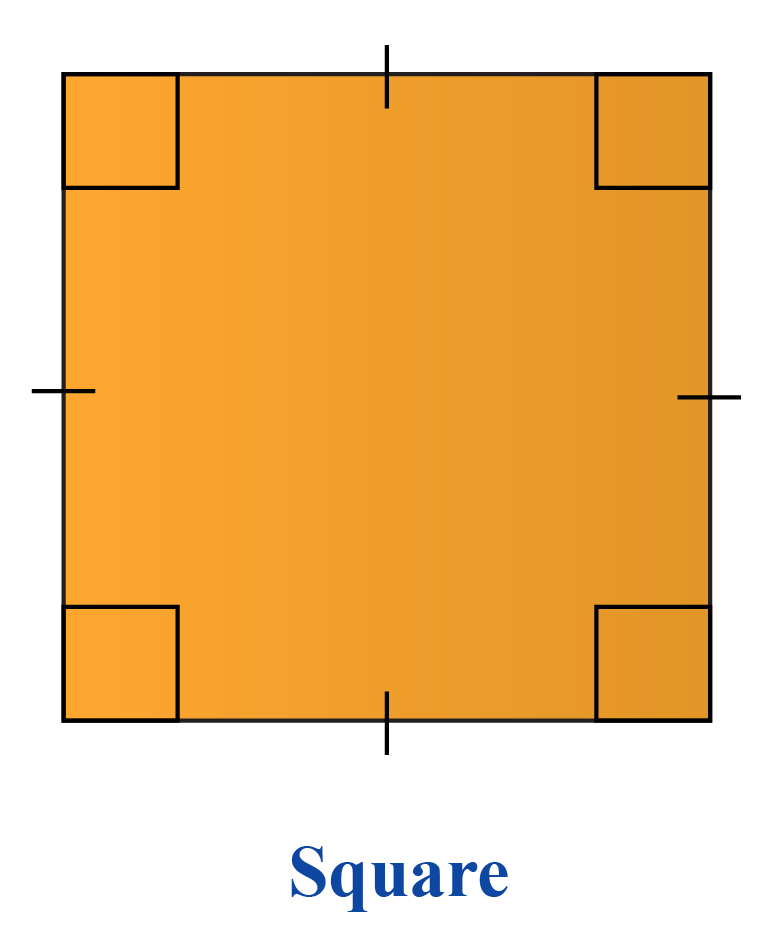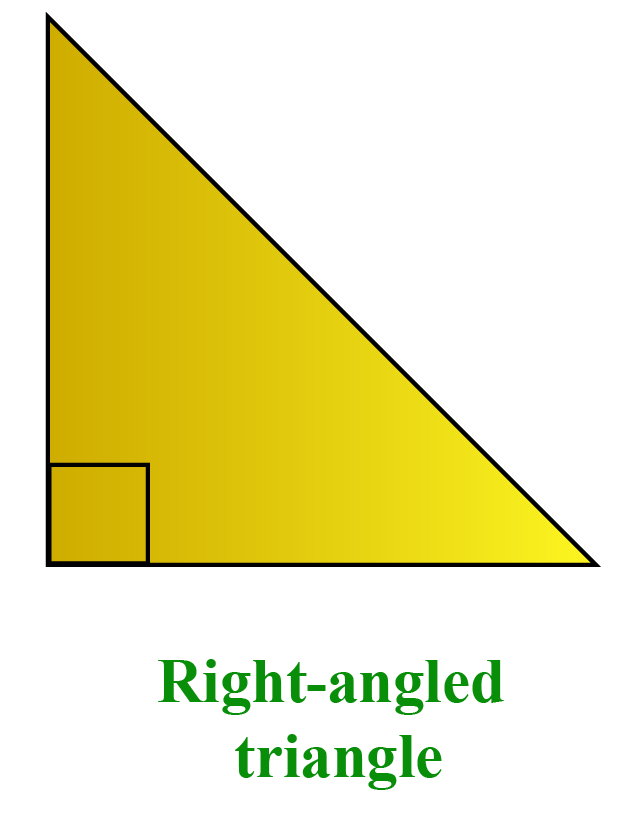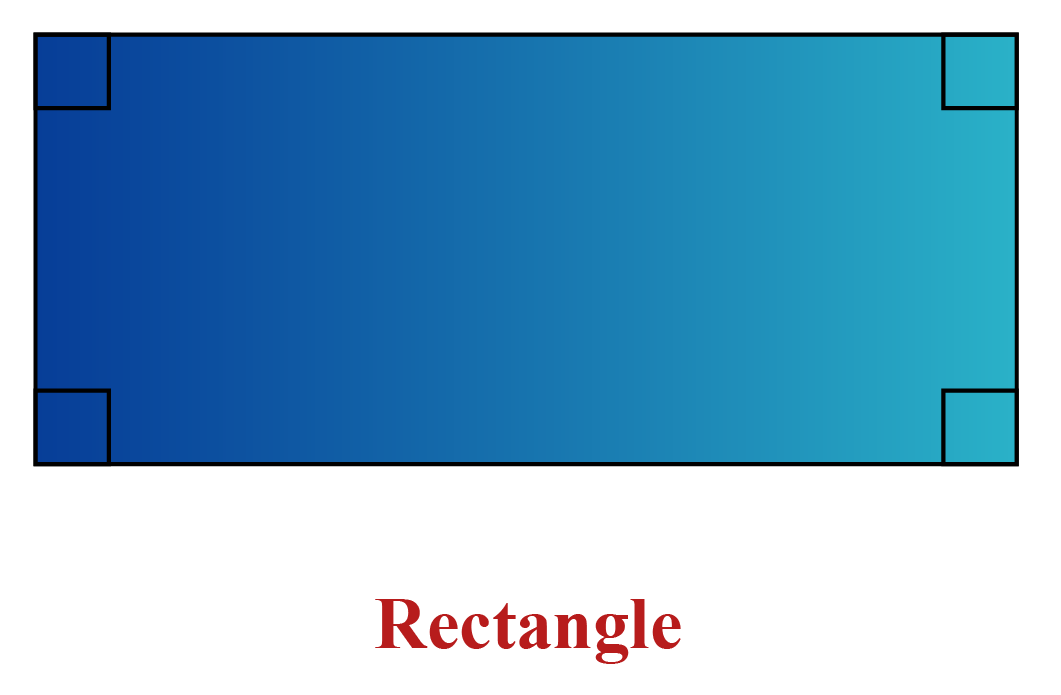Here are some real-life examples of perpendicular lines.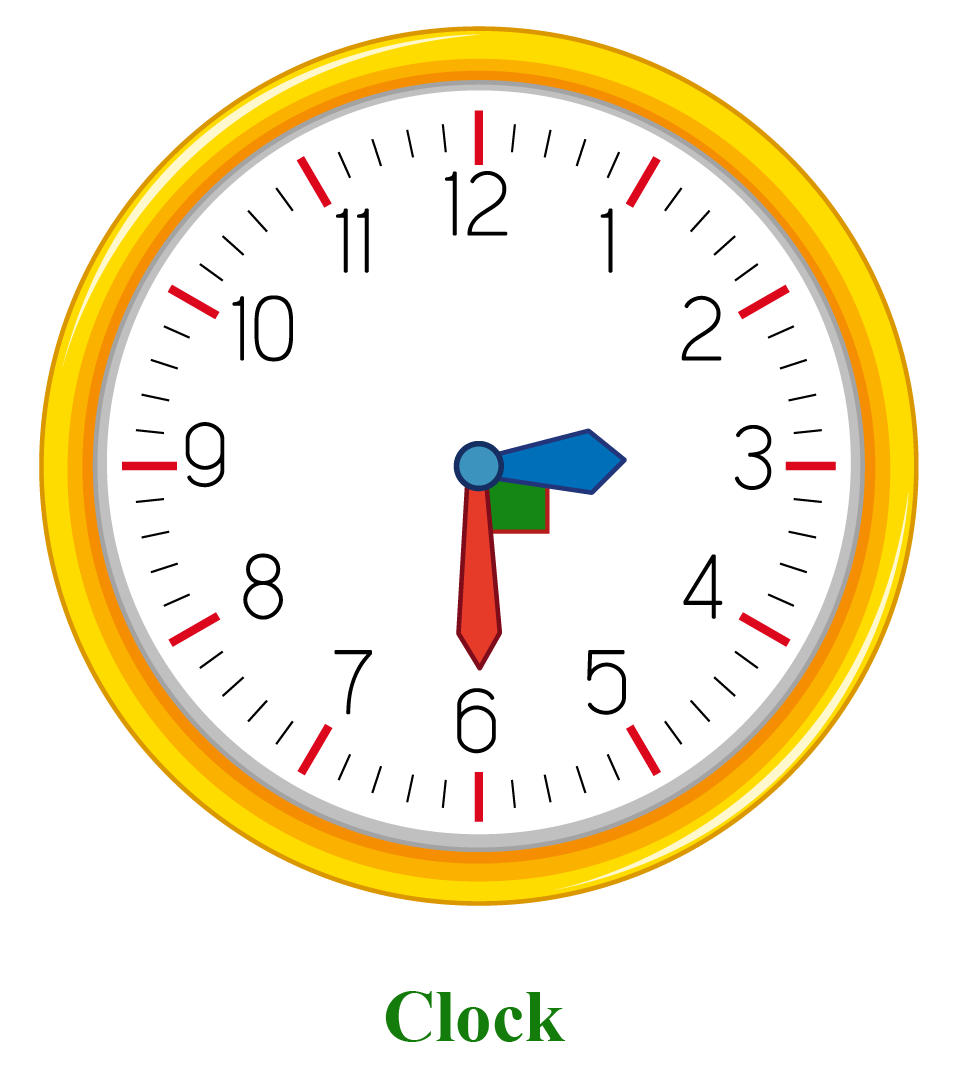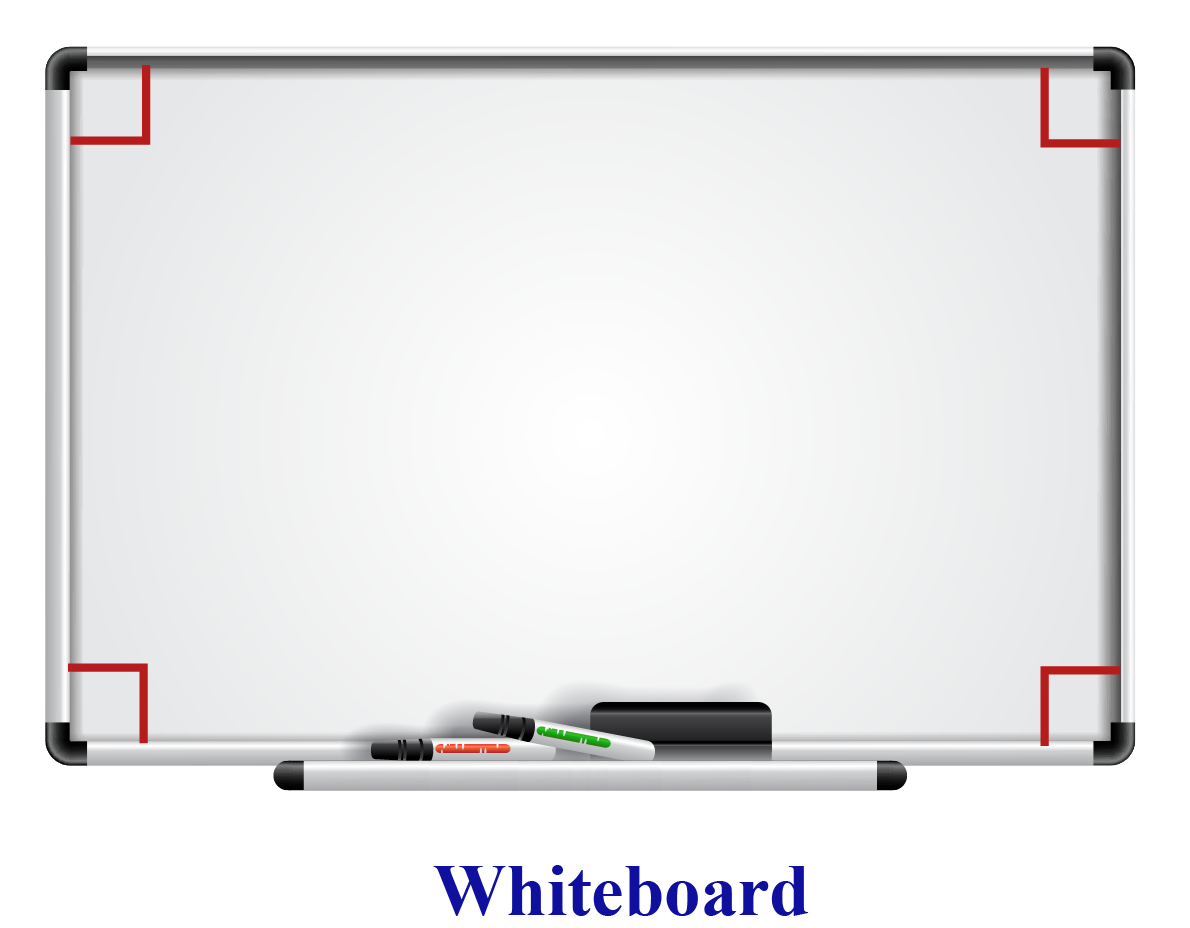You can understand more about perpendicular lines using the following simulation.

Here, you can drag the line $$OA$$ by holding it at $$A$$ and you can see whether $$OA \perp OB$$.Tips and Tricks

If there is an "L" shape in a figure, the corresponding angle at the vertex is a right angle.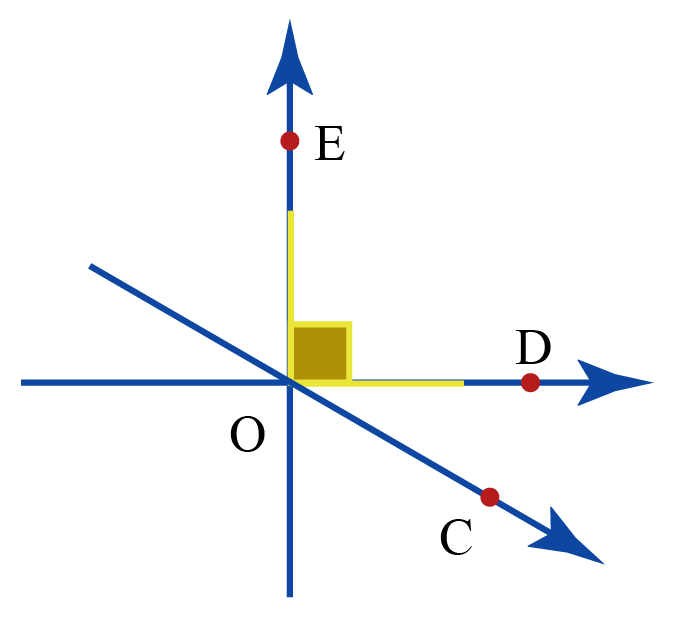## How to Draw Perpendicular Lines?

We can draw a perpendicular line for a given line in two ways.

1. Using protractor
2. Using compass

### Drawing a Perpendicular Using a Protractor

To draw a perpendicular line at a point $$P$$ on a line,

Step 1: Place the baseline of the protractor along the line such that its centre is at $$P$$.

Step 2: Mark a point or a dot $$B$$ at $$90^{\circ}$$ of the protractor.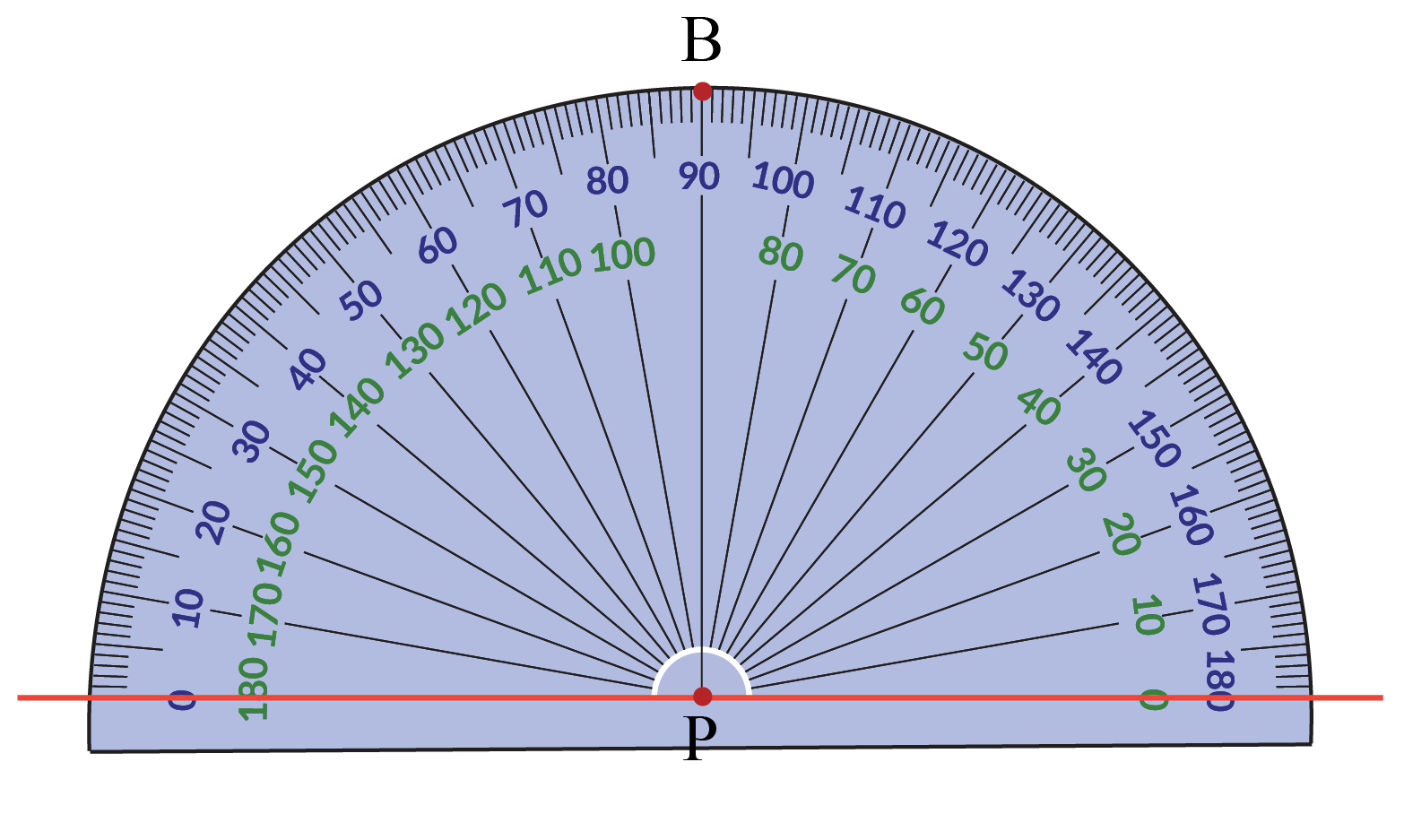Step 3: Remove the protractor and join $$P$$ and $$B$$.

This forms a perpendicular line for the given line.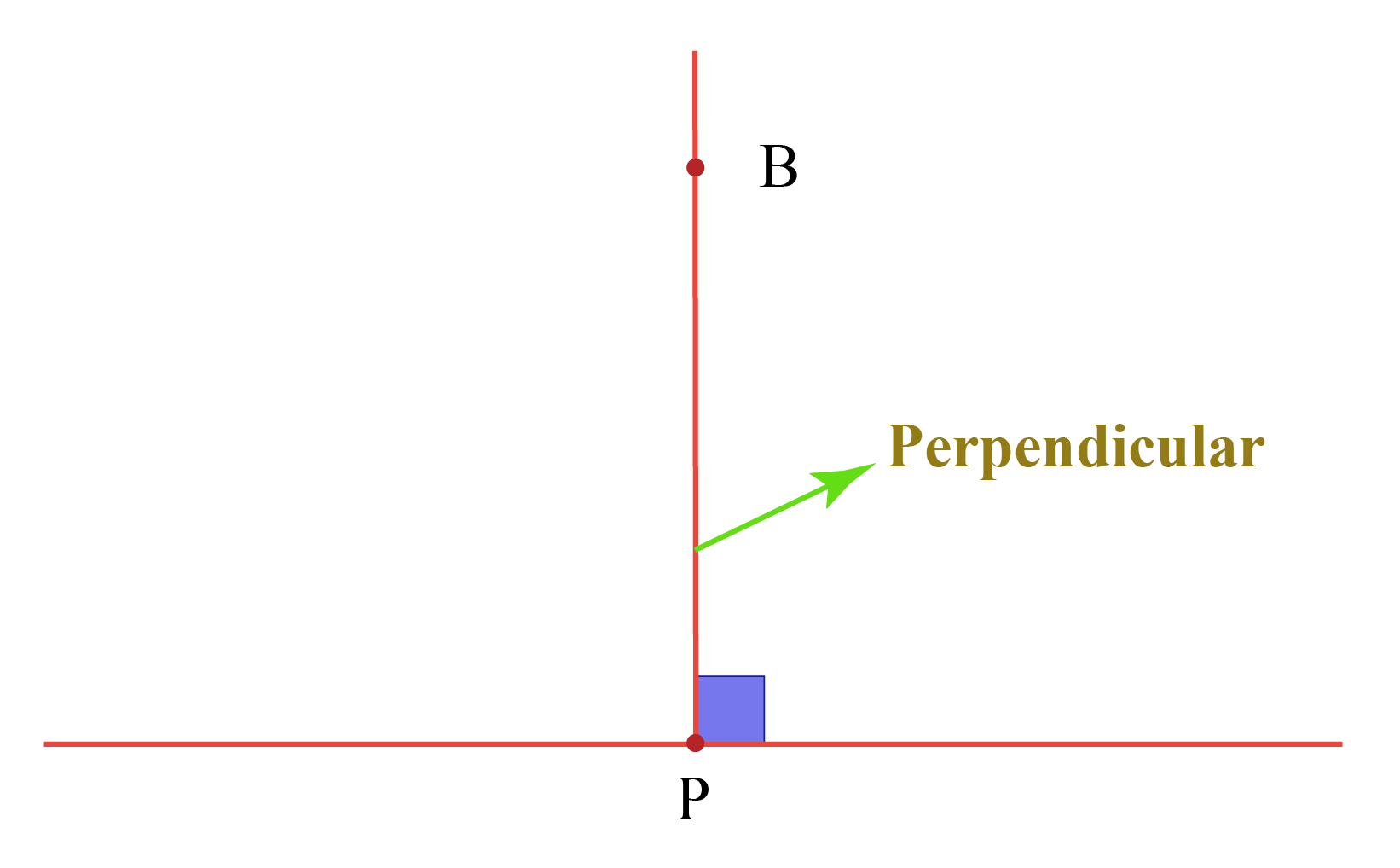### Drawing a Perpendicular Using a Compass

We can draw a perpendicular line without using a protractor but by using a compass instead.

Let's see how.

To draw a perpendicular line at a point $$P$$ on a line,

Step 1: Open the compass to a desired radius.

Step 2: Place the pointer of the compass at $$P$$ and draw a semi-circle which cuts the line at $$A$$ and $$B$$.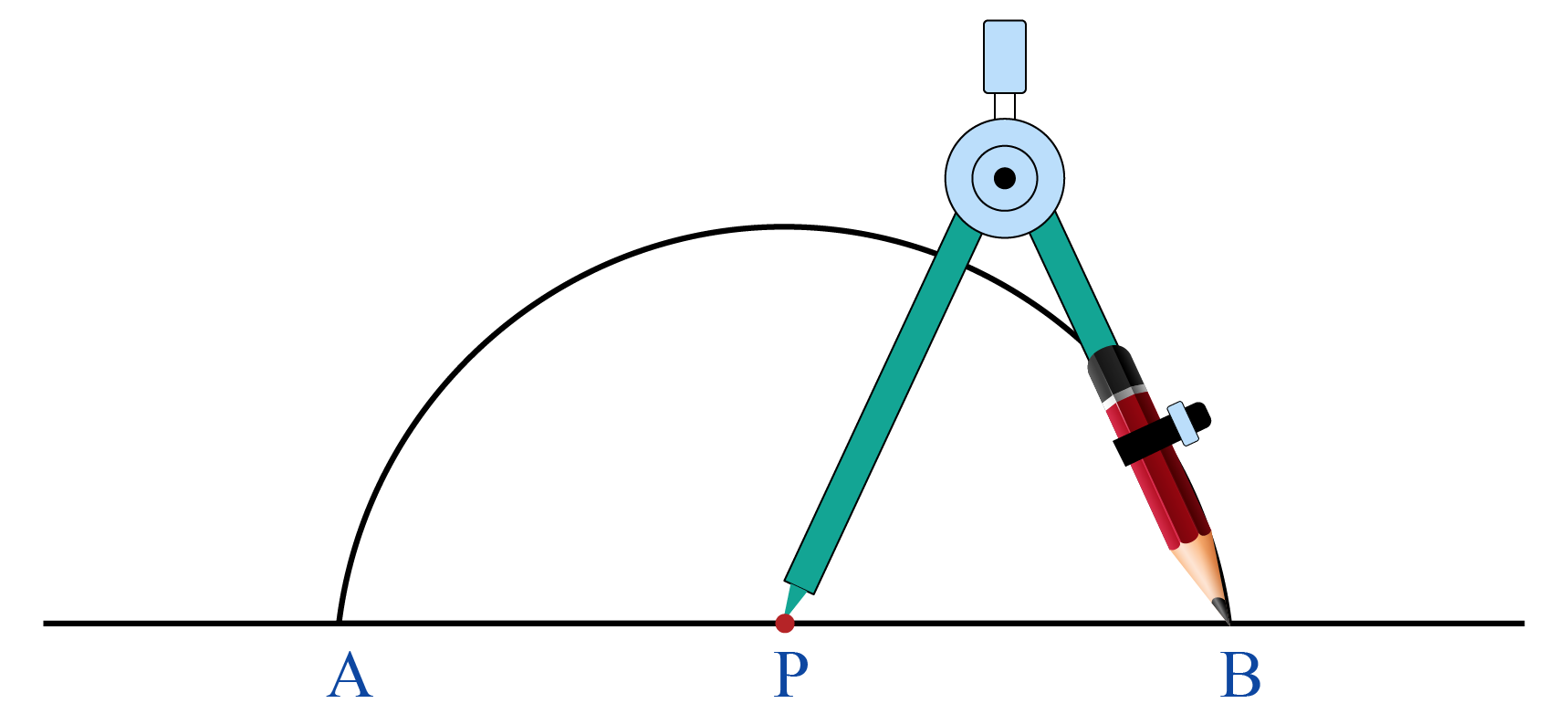Step 3: Without disturbing the radius of the compass, draw two arcs which cut the semi-circle at $$C$$ and $$D$$ by placing the pointer of the compass at $$A$$ and at $$B$$.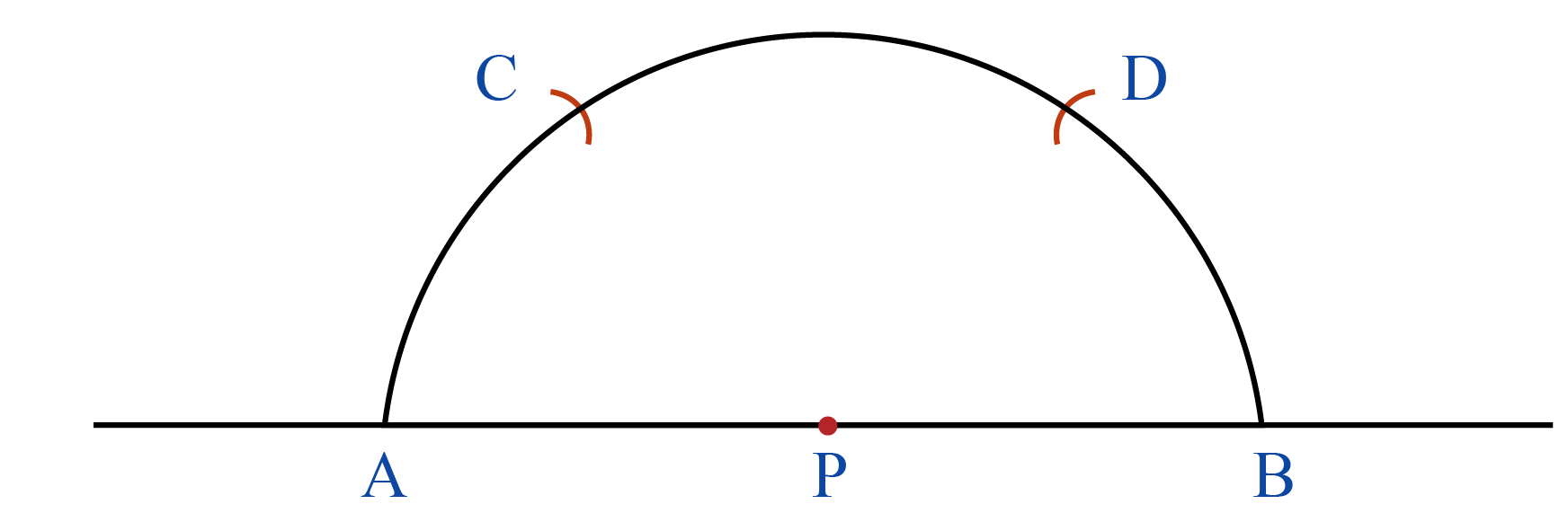Step 4: Without disturbing the radius of the compass, draw two intersecting arcs which intersect at $$Q$$ by placing the pointer of the compass at $$C$$ and at $$D$$.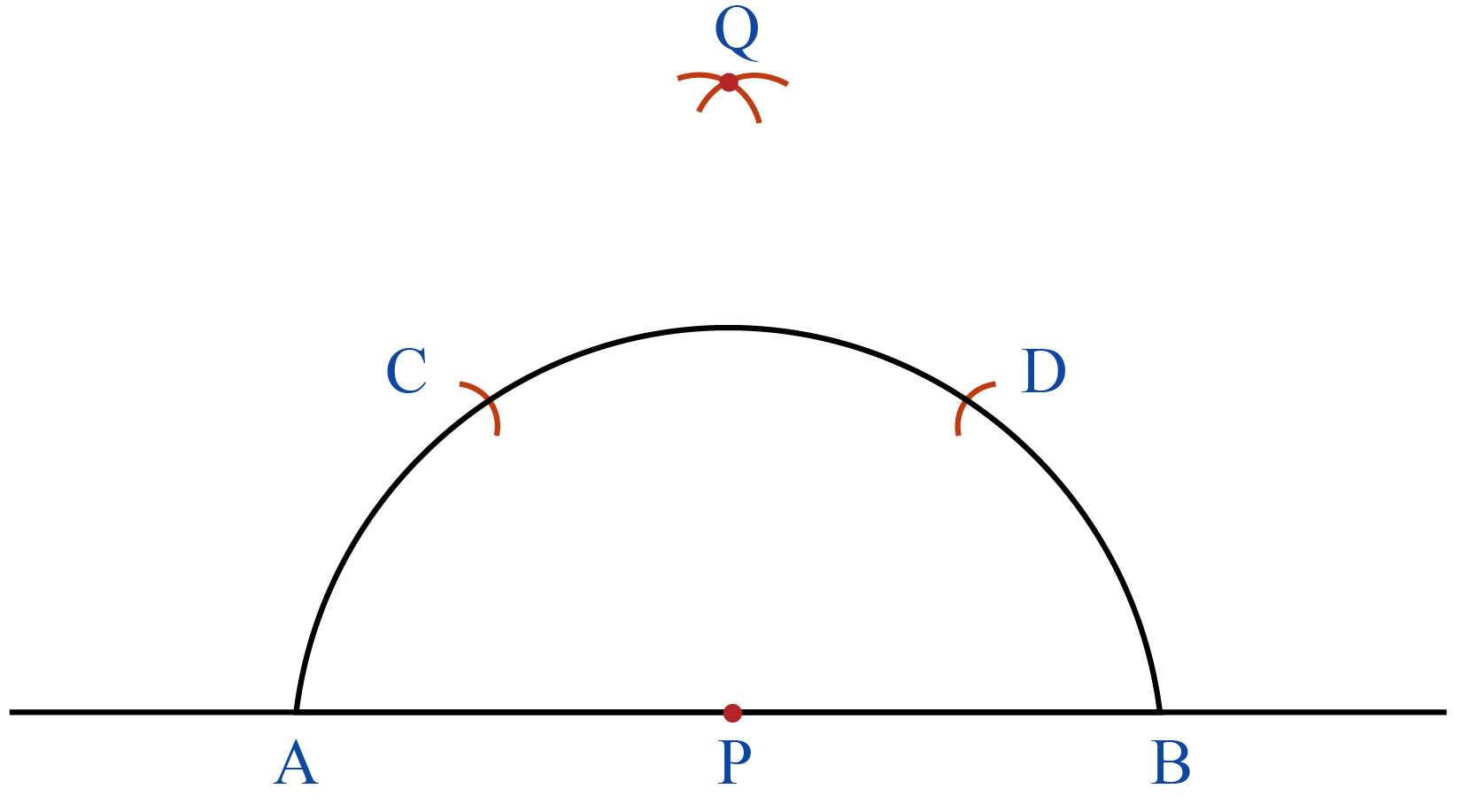Step 5: Join $$P$$ and $$Q$$ which forms a perpendicular line of the given line.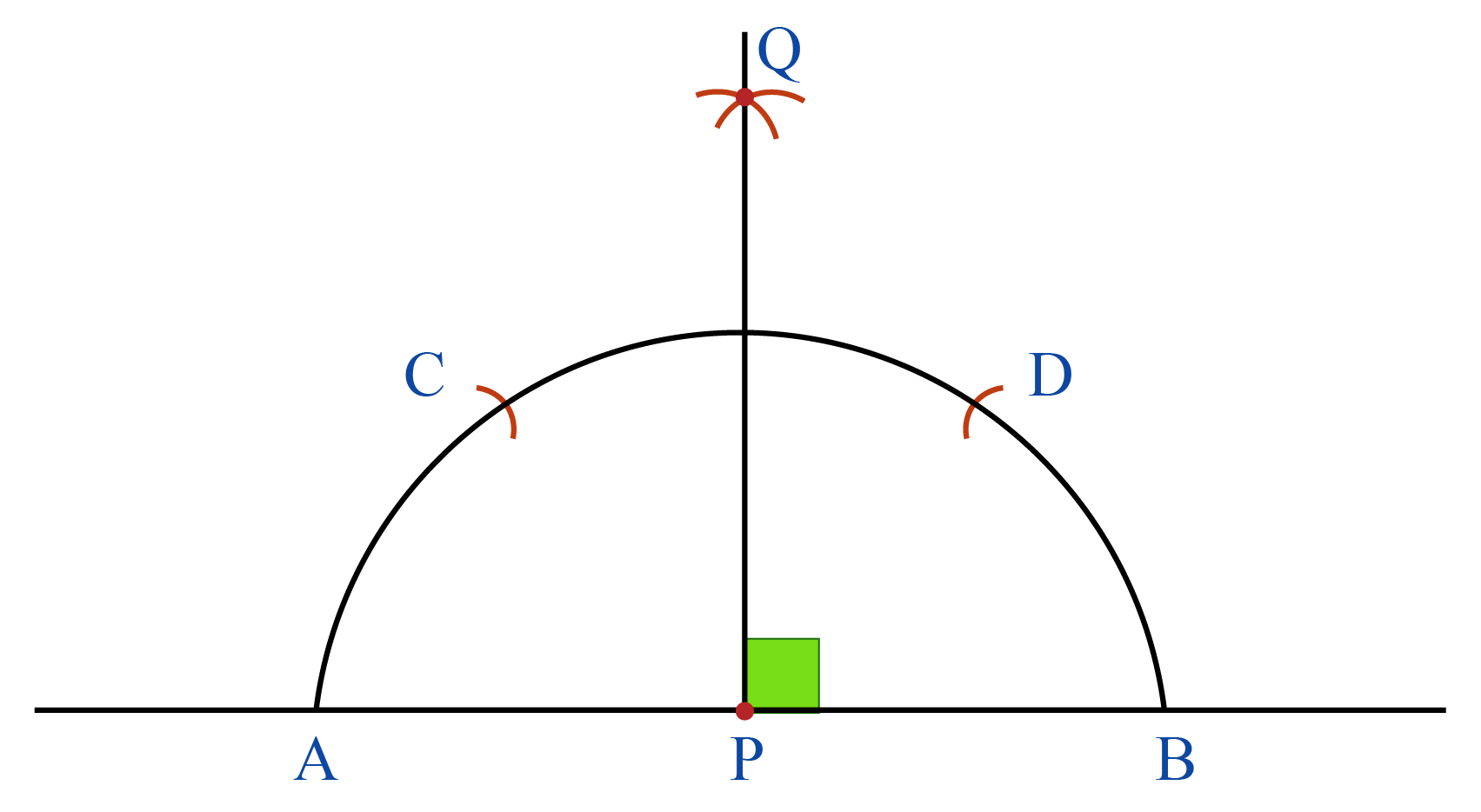## Properties of Perpendicular Lines

There are two important properties of perpendicular lines in math.

• The angle between any two perpendicular lines is always equal to $$90^{\circ}$$
• If two lines are perpendicular to the same line, the two lines are parallel.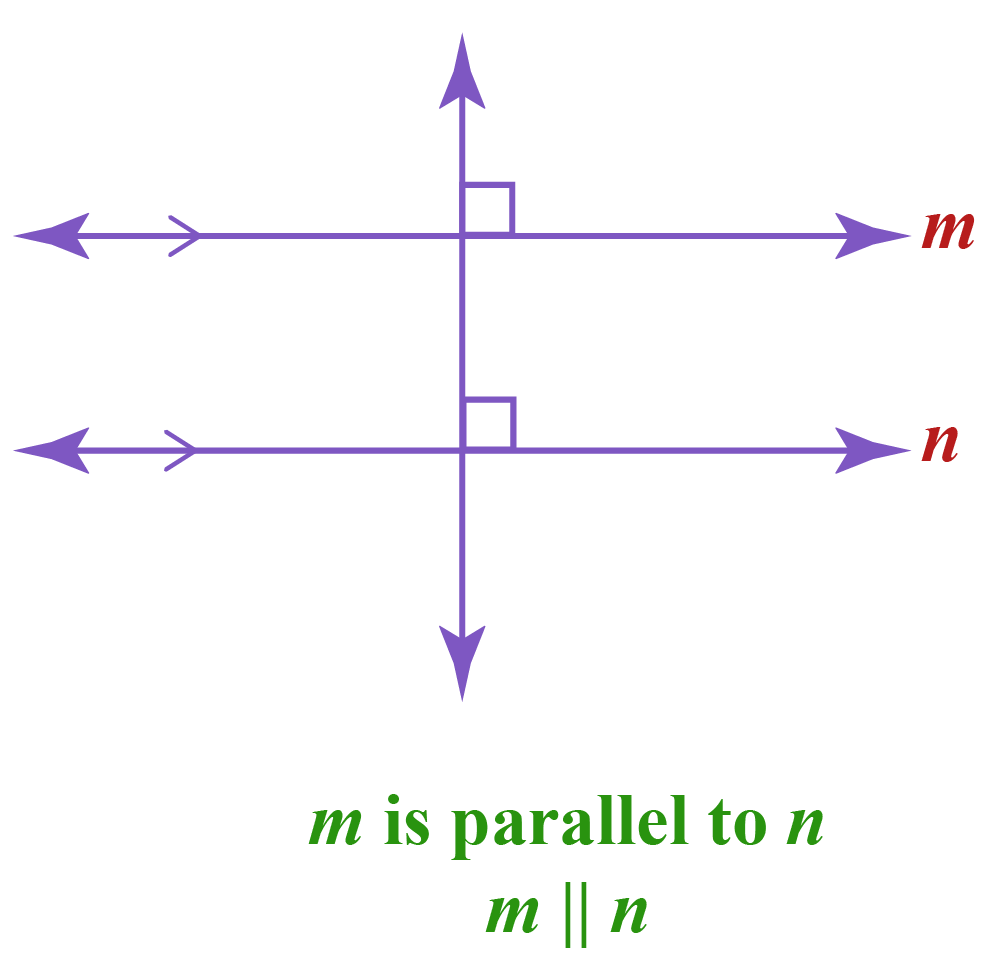Help your child score higher with Cuemath’s proprietary FREE Diagnostic Test. Get access to detailed reports, customized learning plans, and a FREE counseling session. Attempt the test now.

## Solved Examples

 Example 1

Are the hands of the following clock perpendicular to each other?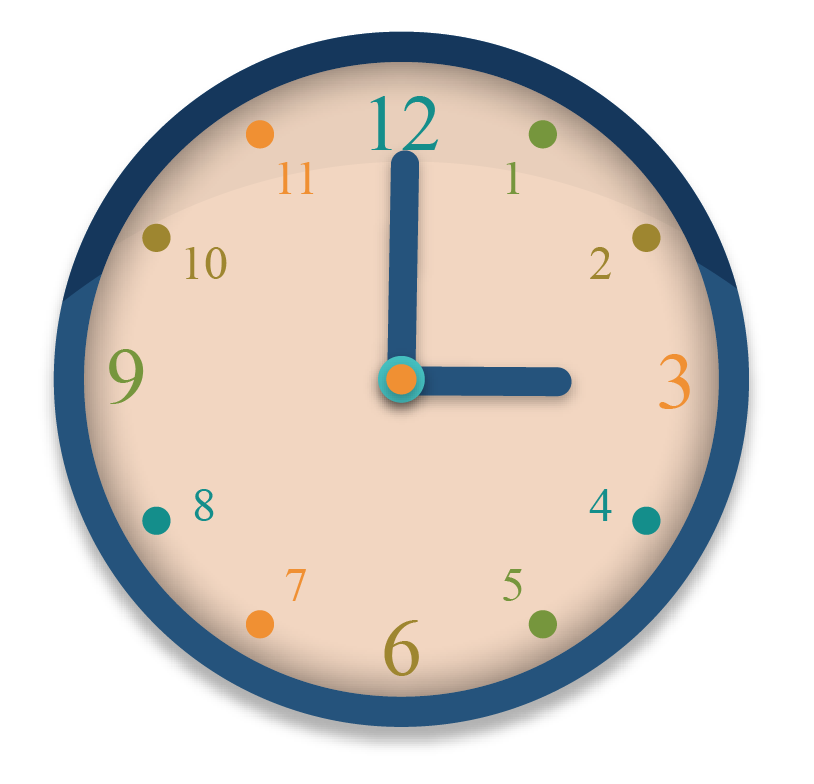Solution:

Place the centre of the protractor at the intersecting point of the hands of the clock.

The baseline of the protractor should be placed along one of the hands of the clock.

Next, measure the angle.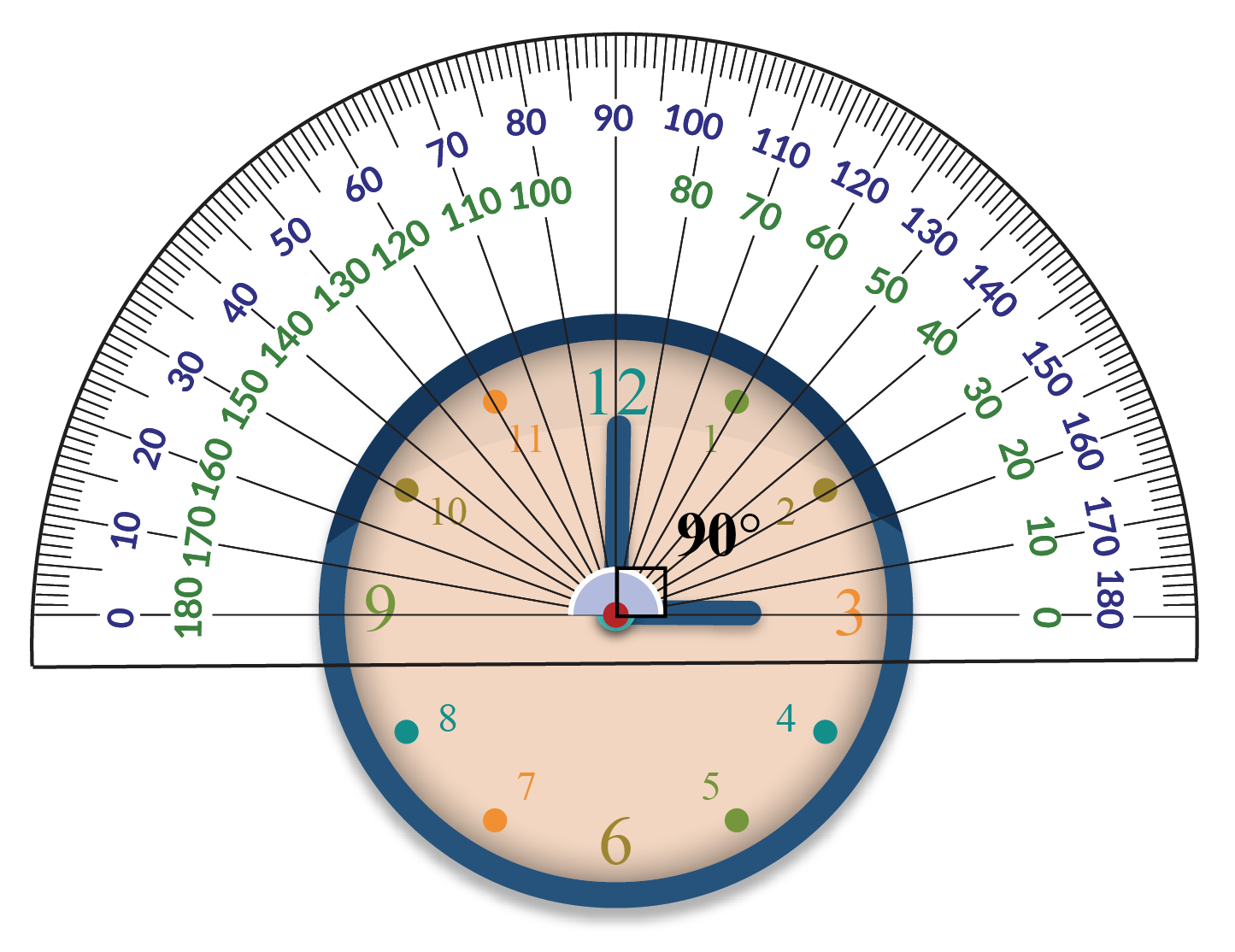Since the angle between the hands of the clock is $$90^{\circ}$$, they are perpendicular.

 $$\therefore$$  The hands are perpendicular
 Example 2

In the following figure, $$AB \perp CD$$.

If $$\angle BOC=90^{\circ}$$, then find the value of $$x$$.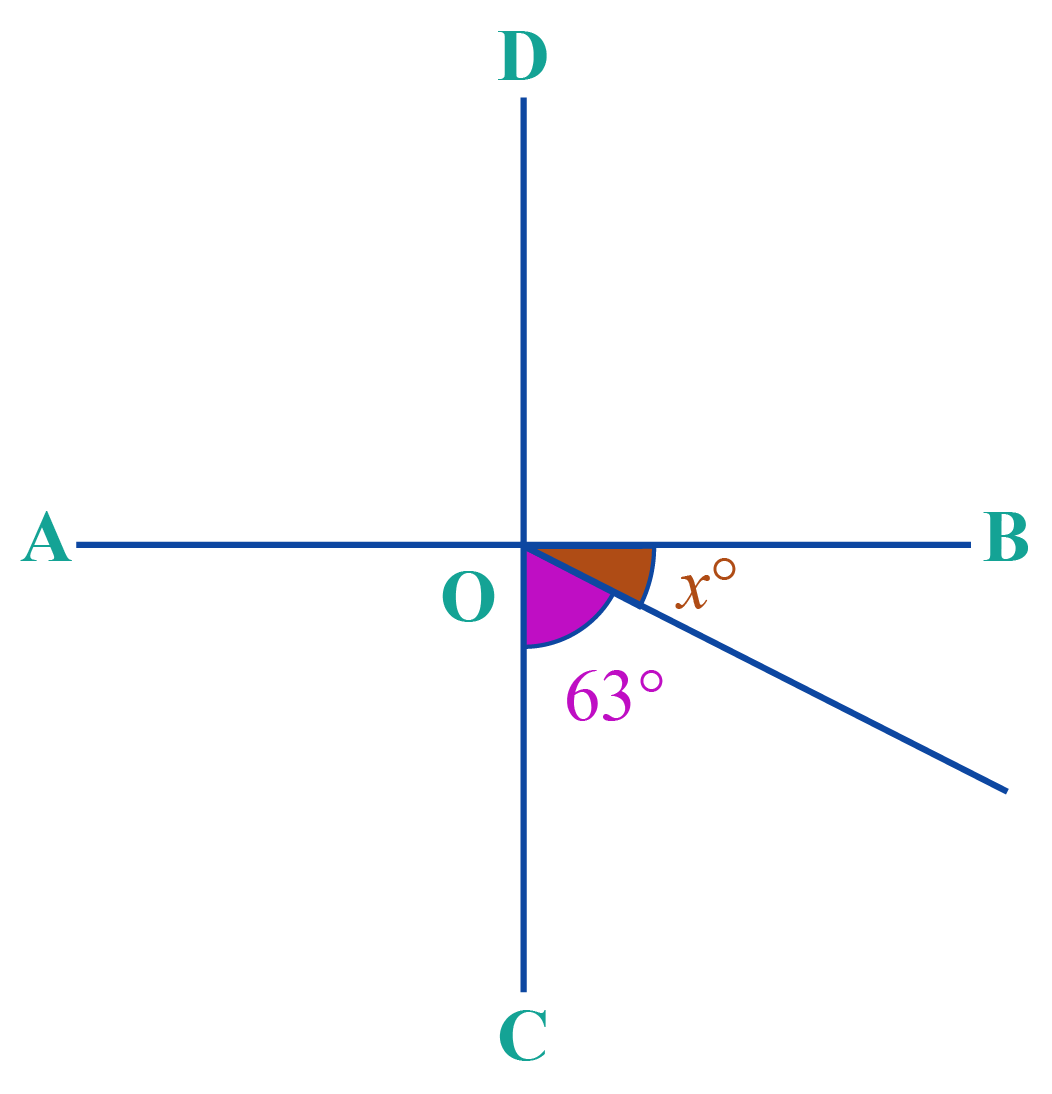Solution:

Since $$\angle BOC=90^{\circ}$$,

\begin{align} x+63&=90\\x &=27 \end{align}

 $x=27$Challenging Questions

Find $$x$$ in the following: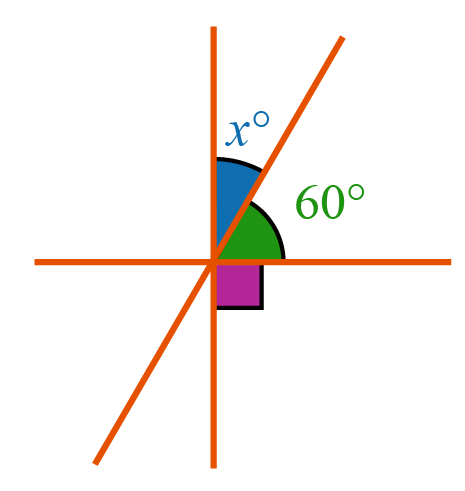CLUEless in Math? Check out how CUEMATH Teachers will explain Perpendicular Line to your kid using interactive simulations & worksheets so they never have to memorise anything in Math again!

Explore Cuemath Live, Interactive & Personalised Online Classes to make your kid a Math Expert. Book a FREE trial class today!

## Practice Questions

Here are a few problems for you to practice. Select/Type your answer and click the "Check Answer" button to see the result.

## Maths Olympiad Sample Papers

IMO (International Maths Olympiad) is a competitive exam in Mathematics conducted annually for school students. It encourages children to develop their math solving skills from a competition perspective.

You can download the FREE grade-wise sample papers from below:

To know more about the Maths Olympiad you can click here

## 1. Do perpendicular lines have to be 90 degrees?

Yes, the angle between two perpendicular lines must be $$90^{\circ}$$

## 2. What is the difference between parallel and perpendicular?

The angle between two parallel lines is $$0^{\circ}$$

The angle between two perpendicular lines is $$90^{\circ}$$

## 3. Does perpendicular mean straight?

No, the perpendicular lines form an "L" shape.

You can learn more about perpendicular lines under the "Definition of Perpendicular" section of this page.

## 4. What is the perpendicular symbol?

The symbol used in between the two lines to say that they are perpendicular is $$\mathbf{\perp}$$

Download Triangles and Quadrilaterals Worksheets
Triangles and Quadrilaterals
grade 9 | Questions Set 1
Triangles and Quadrilaterals
grade 9 | Answers Set 1
Triangles and Quadrilaterals
grade 9 | Questions Set 2
Triangles and Quadrilaterals
grade 9 | Answers Set 2
More Important Topics
Numbers
Algebra
Geometry
Measurement
Money
Data
Trigonometry
Calculus